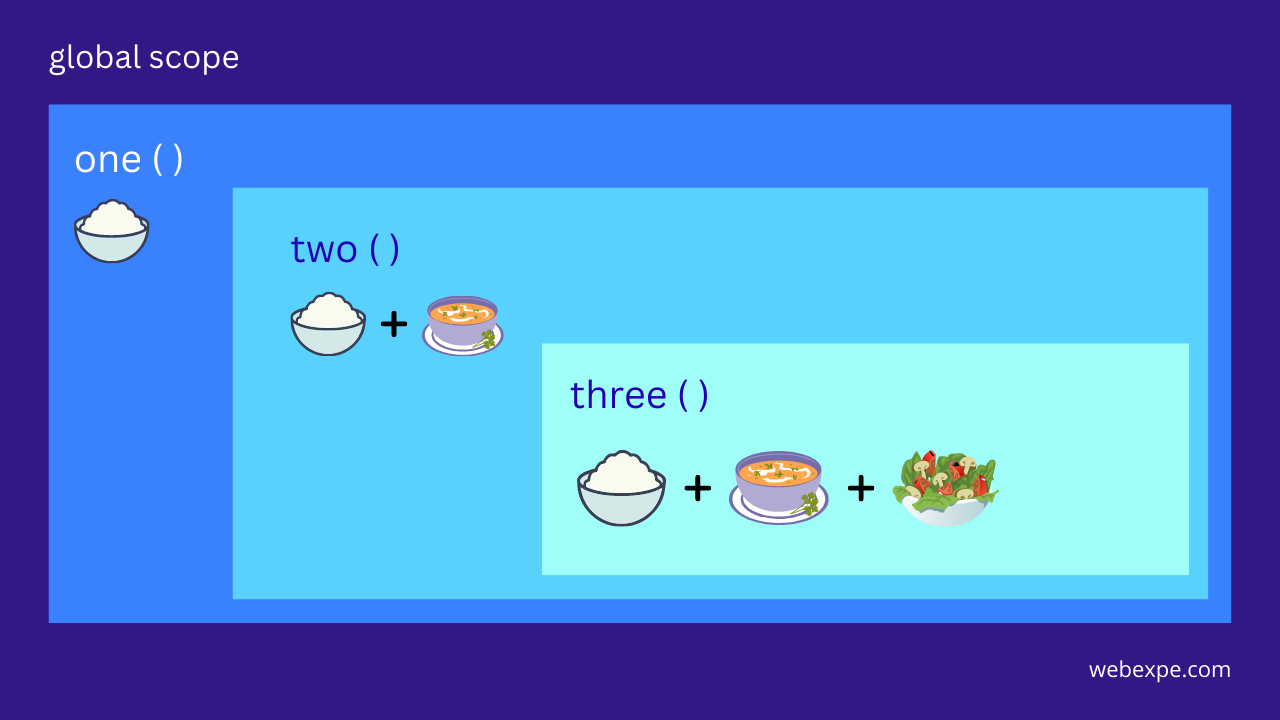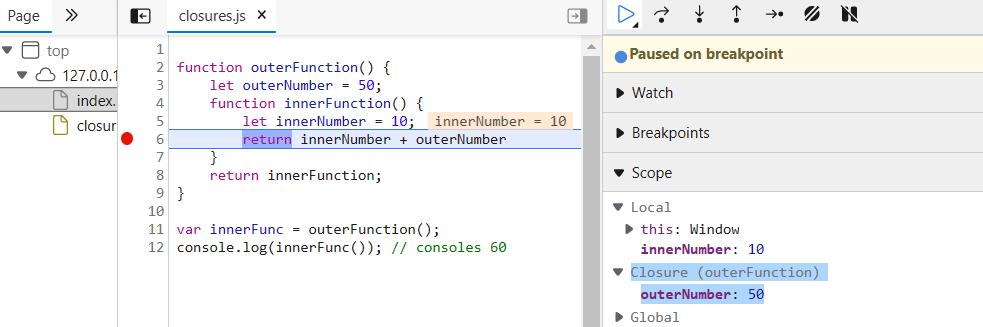# Understanding Closure in JavaScript | Javascript Interview Question

As a web developer, you must have come across the term "closure" many times in JavaScript. However, it's not always clear what it means or how it works. In this article we are going to see concept of closure in JavaScript in a simple and easy-to-understand manner.

Closures work around the concept of a scope. So, first let's see more on how scope works.

## What is Scope?

A scope basically refers to the current context, which determines the accessibility of variables in a program. Every function call creates a new scope. When the function has finished the execution, the scope is usually destroyed.

There are two types of scope global and local. Let's have a look at the below example to understand nested functions and scope.

``````function one() {
const rice = "rice";
const plate = rice;

function two() {
const gravy = "gravy";
const plate = rice + gravy;

function three() {
const plate = rice + gravy + salad;
return plate;
}

return three();
}
return two();
}
const plate = one();
console.log(plate);``````
• Function three will have access to the variables declared in function two and one.
• Function two will have access to the variables declared in function one.

But as functions cannot access the variables declared in it's child/inner functions

• function two cannot access variable of function three
• function one cannot access the variables of both two and three.

When function one is invoked a new scope is created and same is done when function two and three are invoked. once function three has completed its execution its scope will be destroyed, and control will return to function two. once two is done with its execution its scope will also be destroyed and control will be back to function one and the scope of one is destroyed when function one returns a value to variable plate.

The same can be explained with the help of below illustration.Now that we are good with scopes, let's dive right into Closures.

## What is Closure in JavaScript?

Closure is a feature in JavaScript that allows a function to access and use variables from its outer scope even after the outer function has returned. In simple words, it is a function along with all the variables that are accessible in the function's scope. In other words, a closure gives you access to an outer function’s scope inside an inner function.

To understand how closure works, let's consider the following example:

``````function outerFunction() {
let outerNumber = 50;

function innerFunction() {
let innerNumber = 10;
return innerNumber + outerNumber;
}

return innerFunction;
}

var innerFunc = outerFunction();
console.log(innerFunc()); // consoles 60``````

In this example, outerFunction creates a variable called outerNumber and then defines an inner function called innerFunction, which simply returns innerNumber + outerNumber. The outer function then returns the inner function.

When we call outerFunction, it returns the innerFunction, which we store in innerFunc variable. We can then call innerFunc as a regular function, even though it's no longer inside the outerFunction (we are calling it outside of the outerFunction).

When we invoked innerFunc which ultimately invoked innerFunction a closure is formed as seen in the image below. This proves that innerFunc was able to access the outerNumber variable from the outer function because of the closure that is formed.## Bonus! let's consider one more example.

``````const getDescription = (name, birthDate) => {
const calculateAge = () => {
return Math.floor(
(new Date() - new Date(birthDate).getTime()) / 3.15576e10
);
};
return "My name is " + name + ". My age is " + calculateAge();
};

const description = getDescription("John", "1990-10-02");
console.log(description); // "My name is John. My age is 32"``````

In the above code, we can see calculateAge (child function) is invoked from getDescription (parent function). Once calculateAge is invoked we can see in the below image a closure is formed with function getDescription and variable birthDate.1. ## Closures maintain their lexical scope

``````const getInfo = () => {
const birthDate = "2004-11-12";
const calculateAge = () => {
console.log(
Math.floor((new Date() - new Date(birthDate).getTime()) / 3.15576e10)
);
};
return calculateAge;
};

const info = getInfo();
console.log(info()); // 17``````

In the above code snippet when we call that returned function, we can see there was no error as it still had access to const birthDate and logs 17 in the console. That is because after the execution of getInfo is done, its scope is not destroyed in this case.

2. ## Creating private variables

Closures help us create private variables which can help to protect sensitive data and prevent other parts of your code from modifying or accessing it.

3. ## Improving performance

Closure can help improve the performance of your code by reducing the number of global variables and functions that need to be created.

## Conslusion

In conclusion, closures are a powerful and important concept in JavaScript programming. Understanding how they work and how to use them effectively can greatly enhance the functionality and efficiency of your code.

closure
lexical scope
pass by value javaScript